[Updated on 2020-09-03: Updated the algorithm of SARSA and Q-learning so that the difference is more pronounced.
[Updated on 2021-09-19: Thanks to 爱吃猫的鱼, we have this post in Chinese].

A couple of exciting news in Artificial Intelligence (AI) has just happened in recent years. AlphaGo defeated the best professional human player in the game of Go. Very soon the extended algorithm AlphaGo Zero beat AlphaGo by 100-0 without supervised learning on human knowledge. Top professional game players lost to the bot developed by OpenAI on DOTA2 1v1 competition. After knowing these, it is pretty hard not to be curious about the magic behind these algorithms — Reinforcement Learning (RL). I’m writing this post to briefly go over the field. We will first introduce several fundamental concepts and then dive into classic approaches to solving RL problems. Hopefully, this post could be a good starting point for newbies, bridging the future study on the cutting-edge research.

# What is Reinforcement Learning?#

Say, we have an agent in an unknown environment and this agent can obtain some rewards by interacting with the environment. The agent ought to take actions so as to maximize cumulative rewards. In reality, the scenario could be a bot playing a game to achieve high scores, or a robot trying to complete physical tasks with physical items; and not just limited to these.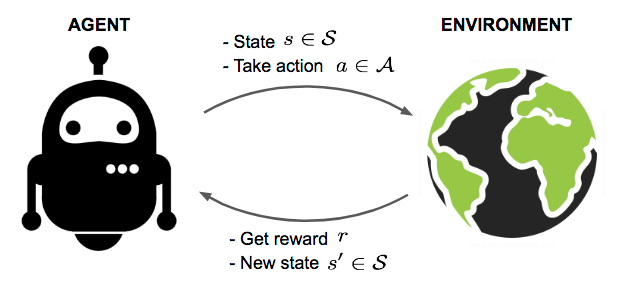The goal of Reinforcement Learning (RL) is to learn a good strategy for the agent from experimental trials and relative simple feedback received. With the optimal strategy, the agent is capable to actively adapt to the environment to maximize future rewards.

## Key Concepts#

Now Let’s formally define a set of key concepts in RL.

The agent is acting in an environment. How the environment reacts to certain actions is defined by a model which we may or may not know. The agent can stay in one of many states ($s \in \mathcal{S}$) of the environment, and choose to take one of many actions ($a \in \mathcal{A}$) to switch from one state to another. Which state the agent will arrive in is decided by transition probabilities between states ($P$). Once an action is taken, the environment delivers a reward ($r \in \mathcal{R}$) as feedback.

The model defines the reward function and transition probabilities. We may or may not know how the model works and this differentiate two circumstances:

• Know the model: planning with perfect information; do model-based RL. When we fully know the environment, we can find the optimal solution by Dynamic Programming (DP). Do you still remember “longest increasing subsequence” or “traveling salesmen problem” from your Algorithms 101 class? LOL. This is not the focus of this post though.
• Does not know the model: learning with incomplete information; do model-free RL or try to learn the model explicitly as part of the algorithm. Most of the following content serves the scenarios when the model is unknown.

The agent’s policy $\pi(s)$ provides the guideline on what is the optimal action to take in a certain state with the goal to maximize the total rewards. Each state is associated with a value function $V(s)$ predicting the expected amount of future rewards we are able to receive in this state by acting the corresponding policy. In other words, the value function quantifies how good a state is. Both policy and value functions are what we try to learn in reinforcement learning.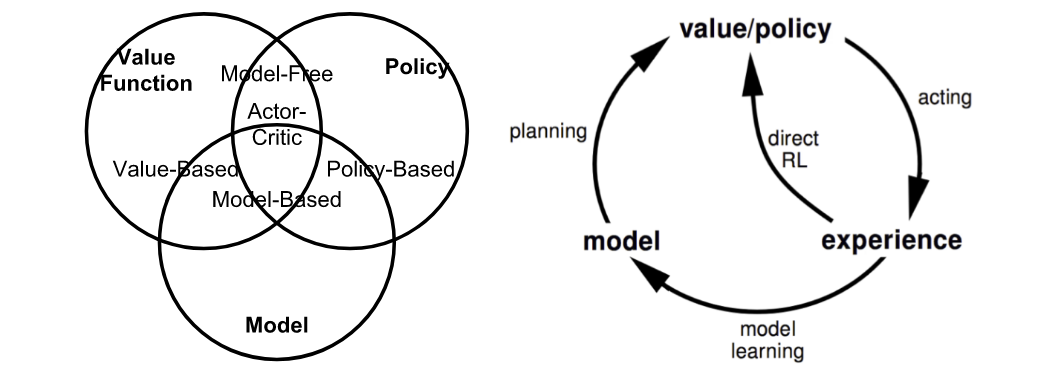The interaction between the agent and the environment involves a sequence of actions and observed rewards in time, $t=1, 2, \dots, T$. During the process, the agent accumulates the knowledge about the environment, learns the optimal policy, and makes decisions on which action to take next so as to efficiently learn the best policy. Let’s label the state, action, and reward at time step t as $S_t$, $A_t$, and $R_t$, respectively. Thus the interaction sequence is fully described by one episode (also known as “trial” or “trajectory”) and the sequence ends at the terminal state $S_T$:

$$S_1, A_1, R_2, S_2, A_2, \dots, S_T$$

Terms you will encounter a lot when diving into different categories of RL algorithms:

• Model-based: Rely on the model of the environment; either the model is known or the algorithm learns it explicitly.
• Model-free: No dependency on the model during learning.
• On-policy: Use the deterministic outcomes or samples from the target policy to train the algorithm.
• Off-policy: Training on a distribution of transitions or episodes produced by a different behavior policy rather than that produced by the target policy.

### Model: Transition and Reward#

The model is a descriptor of the environment. With the model, we can learn or infer how the environment would interact with and provide feedback to the agent. The model has two major parts, transition probability function $P$ and reward function $R$.

Let’s say when we are in state s, we decide to take action a to arrive in the next state s' and obtain reward r. This is known as one transition step, represented by a tuple (s, a, s', r).

The transition function P records the probability of transitioning from state s to s' after taking action a while obtaining reward r. We use $\mathbb{P}$ as a symbol of “probability”.

$$P(s', r \vert s, a) = \mathbb{P} [S_{t+1} = s', R_{t+1} = r \vert S_t = s, A_t = a]$$

Thus the state-transition function can be defined as a function of $P(s', r \vert s, a)$:

$$P_{ss'}^a = P(s' \vert s, a) = \mathbb{P} [S_{t+1} = s' \vert S_t = s, A_t = a] = \sum_{r \in \mathcal{R}} P(s', r \vert s, a)$$

The reward function R predicts the next reward triggered by one action:

$$R(s, a) = \mathbb{E} [R_{t+1} \vert S_t = s, A_t = a] = \sum_{r\in\mathcal{R}} r \sum_{s' \in \mathcal{S}} P(s', r \vert s, a)$$

### Policy#

Policy, as the agent’s behavior function $\pi$, tells us which action to take in state s. It is a mapping from state s to action a and can be either deterministic or stochastic:

• Deterministic: $\pi(s) = a$.
• Stochastic: $\pi(a \vert s) = \mathbb{P}_\pi [A=a \vert S=s]$.

### Value Function#

Value function measures the goodness of a state or how rewarding a state or an action is by a prediction of future reward. The future reward, also known as return, is a total sum of discounted rewards going forward. Let’s compute the return $G_t$ starting from time t:

$$G_t = R_{t+1} + \gamma R_{t+2} + \dots = \sum_{k=0}^{\infty} \gamma^k R_{t+k+1}$$

The discounting factor $\gamma \in [0, 1]$ penalize the rewards in the future, because:

• The future rewards may have higher uncertainty; i.e. stock market.
• The future rewards do not provide immediate benefits; i.e. As human beings, we might prefer to have fun today rather than 5 years later ;).
• Discounting provides mathematical convenience; i.e., we don’t need to track future steps forever to compute return.
• We don’t need to worry about the infinite loops in the state transition graph.

The state-value of a state s is the expected return if we are in this state at time t, $S_t = s$:

$$V_{\pi}(s) = \mathbb{E}_{\pi}[G_t \vert S_t = s]$$

Similarly, we define the action-value (“Q-value”; Q as “Quality” I believe?) of a state-action pair as:

$$Q_{\pi}(s, a) = \mathbb{E}_{\pi}[G_t \vert S_t = s, A_t = a]$$

Additionally, since we follow the target policy $\pi$, we can make use of the probility distribution over possible actions and the Q-values to recover the state-value:

$$V_{\pi}(s) = \sum_{a \in \mathcal{A}} Q_{\pi}(s, a) \pi(a \vert s)$$

The difference between action-value and state-value is the action advantage function (“A-value”):

$$A_{\pi}(s, a) = Q_{\pi}(s, a) - V_{\pi}(s)$$

### Optimal Value and Policy#

The optimal value function produces the maximum return:

$$V_{*}(s) = \max_{\pi} V_{\pi}(s), Q_{*}(s, a) = \max_{\pi} Q_{\pi}(s, a)$$

The optimal policy achieves optimal value functions:

$$\pi_{*} = \arg\max_{\pi} V_{\pi}(s), \pi_{*} = \arg\max_{\pi} Q_{\pi}(s, a)$$

And of course, we have $V_{\pi_{*}}(s)=V_{*}(s)$ and $Q_{\pi_{*}}(s, a) = Q_{*}(s, a)$.

## Markov Decision Processes#

In more formal terms, almost all the RL problems can be framed as Markov Decision Processes (MDPs). All states in MDP has “Markov” property, referring to the fact that the future only depends on the current state, not the history:

$$\mathbb{P}[ S_{t+1} \vert S_t ] = \mathbb{P} [S_{t+1} \vert S_1, \dots, S_t]$$

Or in other words, the future and the past are conditionally independent given the present, as the current state encapsulates all the statistics we need to decide the future.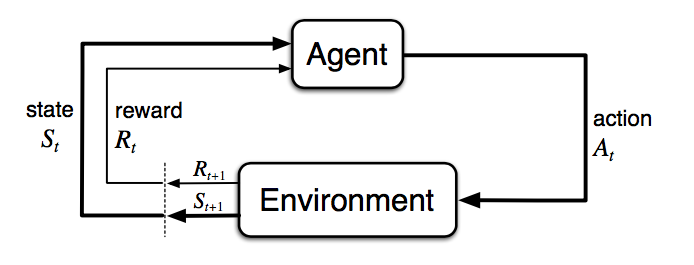A Markov deicison process consists of five elements $\mathcal{M} = \langle \mathcal{S}, \mathcal{A}, P, R, \gamma \rangle$, where the symbols carry the same meanings as key concepts in the previous section, well aligned with RL problem settings:

• $\mathcal{S}$ - a set of states;
• $\mathcal{A}$ - a set of actions;
• $P$ - transition probability function;
• $R$ - reward function;
• $\gamma$ - discounting factor for future rewards. In an unknown environment, we do not have perfect knowledge about $P$ and $R$.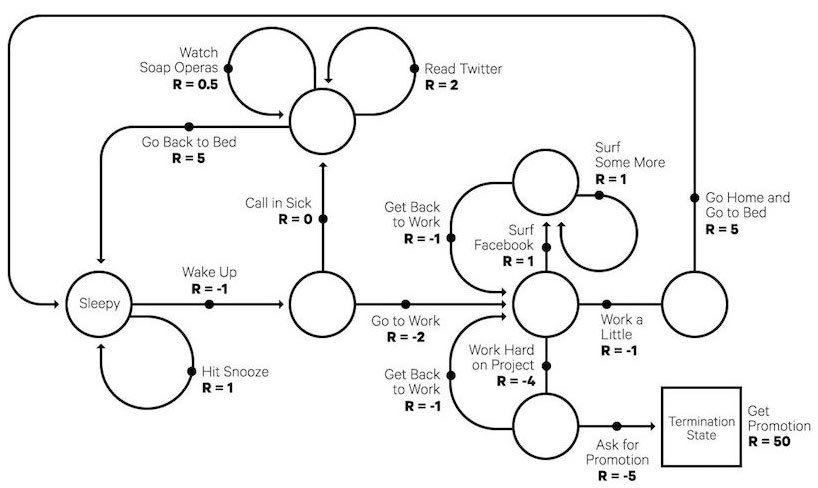## Bellman Equations#

Bellman equations refer to a set of equations that decompose the value function into the immediate reward plus the discounted future values.

\begin{aligned} V(s) &= \mathbb{E}[G_t \vert S_t = s] \\ &= \mathbb{E} [R_{t+1} + \gamma R_{t+2} + \gamma^2 R_{t+3} + \dots \vert S_t = s] \\ &= \mathbb{E} [R_{t+1} + \gamma (R_{t+2} + \gamma R_{t+3} + \dots) \vert S_t = s] \\ &= \mathbb{E} [R_{t+1} + \gamma G_{t+1} \vert S_t = s] \\ &= \mathbb{E} [R_{t+1} + \gamma V(S_{t+1}) \vert S_t = s] \end{aligned}

Similarly for Q-value,

\begin{aligned} Q(s, a) &= \mathbb{E} [R_{t+1} + \gamma V(S_{t+1}) \mid S_t = s, A_t = a] \\ &= \mathbb{E} [R_{t+1} + \gamma \mathbb{E}_{a\sim\pi} Q(S_{t+1}, a) \mid S_t = s, A_t = a] \end{aligned}

### Bellman Expectation Equations#

The recursive update process can be further decomposed to be equations built on both state-value and action-value functions. As we go further in future action steps, we extend V and Q alternatively by following the policy $\pi$.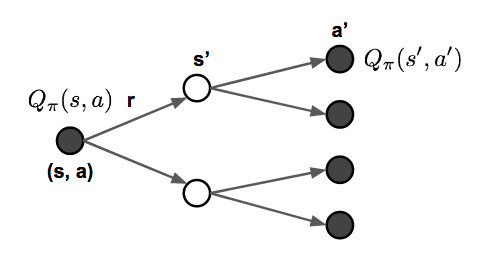\begin{aligned} V_{\pi}(s) &= \sum_{a \in \mathcal{A}} \pi(a \vert s) Q_{\pi}(s, a) \\ Q_{\pi}(s, a) &= R(s, a) + \gamma \sum_{s' \in \mathcal{S}} P_{ss'}^a V_{\pi} (s') \\ V_{\pi}(s) &= \sum_{a \in \mathcal{A}} \pi(a \vert s) \big( R(s, a) + \gamma \sum_{s' \in \mathcal{S}} P_{ss'}^a V_{\pi} (s') \big) \\ Q_{\pi}(s, a) &= R(s, a) + \gamma \sum_{s' \in \mathcal{S}} P_{ss'}^a \sum_{a' \in \mathcal{A}} \pi(a' \vert s') Q_{\pi} (s', a') \end{aligned}

### Bellman Optimality Equations#

If we are only interested in the optimal values, rather than computing the expectation following a policy, we could jump right into the maximum returns during the alternative updates without using a policy. RECAP: the optimal values $V_*$ and $Q_*$ are the best returns we can obtain, defined here.

\begin{aligned} V_*(s) &= \max_{a \in \mathcal{A}} Q_*(s,a)\\ Q_*(s, a) &= R(s, a) + \gamma \sum_{s' \in \mathcal{S}} P_{ss'}^a V_*(s') \\ V_*(s) &= \max_{a \in \mathcal{A}} \big( R(s, a) + \gamma \sum_{s' \in \mathcal{S}} P_{ss'}^a V_*(s') \big) \\ Q_*(s, a) &= R(s, a) + \gamma \sum_{s' \in \mathcal{S}} P_{ss'}^a \max_{a' \in \mathcal{A}} Q_*(s', a') \end{aligned}

Unsurprisingly they look very similar to Bellman expectation equations.

If we have complete information of the environment, this turns into a planning problem, solvable by DP. Unfortunately, in most scenarios, we do not know $P_{ss'}^a$ or $R(s, a)$, so we cannot solve MDPs by directly applying Bellmen equations, but it lays the theoretical foundation for many RL algorithms.

# Common Approaches#

Now it is the time to go through the major approaches and classic algorithms for solving RL problems. In future posts, I plan to dive into each approach further.

## Dynamic Programming#

When the model is fully known, following Bellman equations, we can use Dynamic Programming (DP) to iteratively evaluate value functions and improve policy.

### Policy Evaluation#

Policy Evaluation is to compute the state-value $V_\pi$ for a given policy $\pi$:

$$V_{t+1}(s) = \mathbb{E}_\pi [r + \gamma V_t(s') | S_t = s] = \sum_a \pi(a \vert s) \sum_{s', r} P(s', r \vert s, a) (r + \gamma V_t(s'))$$

### Policy Improvement#

Based on the value functions, Policy Improvement generates a better policy $\pi' \geq \pi$ by acting greedily.

$$Q_\pi(s, a) = \mathbb{E} [R_{t+1} + \gamma V_\pi(S_{t+1}) \vert S_t=s, A_t=a] = \sum_{s', r} P(s', r \vert s, a) (r + \gamma V_\pi(s'))$$

### Policy Iteration#

The Generalized Policy Iteration (GPI) algorithm refers to an iterative procedure to improve the policy when combining policy evaluation and improvement.

$$\pi_0 \xrightarrow[]{\text{evaluation}} V_{\pi_0} \xrightarrow[]{\text{improve}} \pi_1 \xrightarrow[]{\text{evaluation}} V_{\pi_1} \xrightarrow[]{\text{improve}} \pi_2 \xrightarrow[]{\text{evaluation}} \dots \xrightarrow[]{\text{improve}} \pi_* \xrightarrow[]{\text{evaluation}} V_*$$

In GPI, the value function is approximated repeatedly to be closer to the true value of the current policy and in the meantime, the policy is improved repeatedly to approach optimality. This policy iteration process works and always converges to the optimality, but why this is the case?

Say, we have a policy $\pi$ and then generate an improved version $\pi'$ by greedily taking actions, $\pi'(s) = \arg\max_{a \in \mathcal{A}} Q_\pi(s, a)$. The value of this improved $\pi'$ is guaranteed to be better because:

\begin{aligned} Q_\pi(s, \pi'(s)) &= Q_\pi(s, \arg\max_{a \in \mathcal{A}} Q_\pi(s, a)) \\ &= \max_{a \in \mathcal{A}} Q_\pi(s, a) \geq Q_\pi(s, \pi(s)) = V_\pi(s) \end{aligned}

## Monte-Carlo Methods#

First, let’s recall that $V(s) = \mathbb{E}[ G_t \vert S_t=s]$. Monte-Carlo (MC) methods uses a simple idea: It learns from episodes of raw experience without modeling the environmental dynamics and computes the observed mean return as an approximation of the expected return. To compute the empirical return $G_t$, MC methods need to learn from complete episodes $S_1, A_1, R_2, \dots, S_T$ to compute $G_t = \sum_{k=0}^{T-t-1} \gamma^k R_{t+k+1}$ and all the episodes must eventually terminate.

The empirical mean return for state s is:

$$V(s) = \frac{\sum_{t=1}^T \mathbb{1}[S_t = s] G_t}{\sum_{t=1}^T \mathbb{1}[S_t = s]}$$

where $\mathbb{1}[S_t = s]$ is a binary indicator function. We may count the visit of state s every time so that there could exist multiple visits of one state in one episode (“every-visit”), or only count it the first time we encounter a state in one episode (“first-visit”). This way of approximation can be easily extended to action-value functions by counting (s, a) pair.

$$Q(s, a) = \frac{\sum_{t=1}^T \mathbb{1}[S_t = s, A_t = a] G_t}{\sum_{t=1}^T \mathbb{1}[S_t = s, A_t = a]}$$

To learn the optimal policy by MC, we iterate it by following a similar idea to GPI.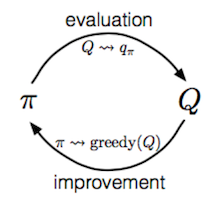1. Improve the policy greedily with respect to the current value function: $\pi(s) = \arg\max_{a \in \mathcal{A}} Q(s, a)$.
2. Generate a new episode with the new policy $\pi$ (i.e. using algorithms like ε-greedy helps us balance between exploitation and exploration.)
3. Estimate Q using the new episode: $q_\pi(s, a) = \frac{\sum_{t=1}^T \big( \mathbb{1}[S_t = s, A_t = a] \sum_{k=0}^{T-t-1} \gamma^k R_{t+k+1} \big)}{\sum_{t=1}^T \mathbb{1}[S_t = s, A_t = a]}$

## Temporal-Difference Learning#

Similar to Monte-Carlo methods, Temporal-Difference (TD) Learning is model-free and learns from episodes of experience. However, TD learning can learn from incomplete episodes and hence we don’t need to track the episode up to termination. TD learning is so important that Sutton & Barto (2017) in their RL book describes it as “one idea … central and novel to reinforcement learning”.

### Bootstrapping#

TD learning methods update targets with regard to existing estimates rather than exclusively relying on actual rewards and complete returns as in MC methods. This approach is known as bootstrapping.

### Value Estimation#

The key idea in TD learning is to update the value function $V(S_t)$ towards an estimated return $R_{t+1} + \gamma V(S_{t+1})$ (known as “TD target"). To what extent we want to update the value function is controlled by the learning rate hyperparameter α:

\begin{aligned} V(S_t) &\leftarrow (1- \alpha) V(S_t) + \alpha G_t \\ V(S_t) &\leftarrow V(S_t) + \alpha (G_t - V(S_t)) \\ V(S_t) &\leftarrow V(S_t) + \alpha (R_{t+1} + \gamma V(S_{t+1}) - V(S_t)) \end{aligned}

Similarly, for action-value estimation:

$$Q(S_t, A_t) \leftarrow Q(S_t, A_t) + \alpha (R_{t+1} + \gamma Q(S_{t+1}, A_{t+1}) - Q(S_t, A_t))$$

Next, let’s dig into the fun part on how to learn optimal policy in TD learning (aka “TD control”). Be prepared, you are gonna see many famous names of classic algorithms in this section.

### SARSA: On-Policy TD control#

“SARSA” refers to the procedure of updaing Q-value by following a sequence of $\dots, S_t, A_t, R_{t+1}, S_{t+1}, A_{t+1}, \dots$. The idea follows the same route of GPI. Within one episode, it works as follows:

1. Initialize $t=0$.
2. Start with $S_0$ and choose action $A_0 = \arg\max_{a \in \mathcal{A}} Q(S_0, a)$, where $\epsilon$-greedy is commonly applied.
3. At time $t$, after applying action $A_t$, we observe reward $R_{t+1}$ and get into the next state $S_{t+1}$.
4. Then pick the next action in the same way as in step 2: $A_{t+1} = \arg\max_{a \in \mathcal{A}} Q(S_{t+1}, a)$.
5. Update the Q-value function: $Q(S_t, A_t) \leftarrow Q(S_t, A_t) + \alpha (R_{t+1} + \gamma Q(S_{t+1}, A_{t+1}) - Q(S_t, A_t))$.
6. Set $t = t+1$ and repeat from step 3.

In each step of SARSA, we need to choose the next action according to the current policy.

### Q-Learning: Off-policy TD control#

The development of Q-learning (Watkins & Dayan, 1992) is a big breakout in the early days of Reinforcement Learning. Within one episode, it works as follows:

1. Initialize $t=0$.
2. Starts with $S_0$.
3. At time step $t$, we pick the action according to Q values, $A_t = \arg\max_{a \in \mathcal{A}} Q(S_t, a)$ and $\epsilon$-greedy is commonly applied.
4. After applying action $A_t$, we observe reward $R_{t+1}$ and get into the next state $S_{t+1}$.
5. Update the Q-value function: $Q(S_t, A_t) \leftarrow Q(S_t, A_t) + \alpha (R_{t+1} + \gamma \max_{a \in \mathcal{A}} Q(S_{t+1}, a) - Q(S_t, A_t))$.
6. $t = t+1$ and repeat from step 3.

The key difference from SARSA is that Q-learning does not follow the current policy to pick the second action $A_{t+1}$. It estimates $Q^*$ out of the best Q values, but which action (denoted as $a^*$) leads to this maximal Q does not matter and in the next step Q-learning may not follow $a^*$.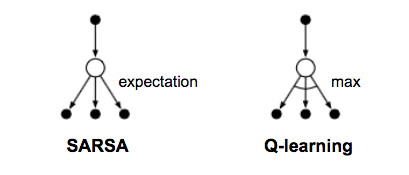### Deep Q-Network#

Theoretically, we can memorize $Q_*(.)$ for all state-action pairs in Q-learning, like in a gigantic table. However, it quickly becomes computationally infeasible when the state and action space are large. Thus people use functions (i.e. a machine learning model) to approximate Q values and this is called function approximation. For example, if we use a function with parameter $\theta$ to calculate Q values, we can label Q value function as $Q(s, a; \theta)$.

Unfortunately Q-learning may suffer from instability and divergence when combined with an nonlinear Q-value function approximation and bootstrapping (See Problems #2).

Deep Q-Network (“DQN”; Mnih et al. 2015) aims to greatly improve and stabilize the training procedure of Q-learning by two innovative mechanisms:

• Experience Replay: All the episode steps $e_t = (S_t, A_t, R_t, S_{t+1})$ are stored in one replay memory $D_t = \{ e_1, \dots, e_t \}$. $D_t$ has experience tuples over many episodes. During Q-learning updates, samples are drawn at random from the replay memory and thus one sample could be used multiple times. Experience replay improves data efficiency, removes correlations in the observation sequences, and smooths over changes in the data distribution.
• Periodically Updated Target: Q is optimized towards target values that are only periodically updated. The Q network is cloned and kept frozen as the optimization target every C steps (C is a hyperparameter). This modification makes the training more stable as it overcomes the short-term oscillations.

The loss function looks like this:

$$\mathcal{L}(\theta) = \mathbb{E}_{(s, a, r, s') \sim U(D)} \Big[ \big( r + \gamma \max_{a'} Q(s', a'; \theta^{-}) - Q(s, a; \theta) \big)^2 \Big]$$

where $U(D)$ is a uniform distribution over the replay memory D; $\theta^{-}$ is the parameters of the frozen target Q-network.

In addition, it is also found to be helpful to clip the error term to be between [-1, 1]. (I always get mixed feeling with parameter clipping, as many studies have shown that it works empirically but it makes the math much less pretty. :/)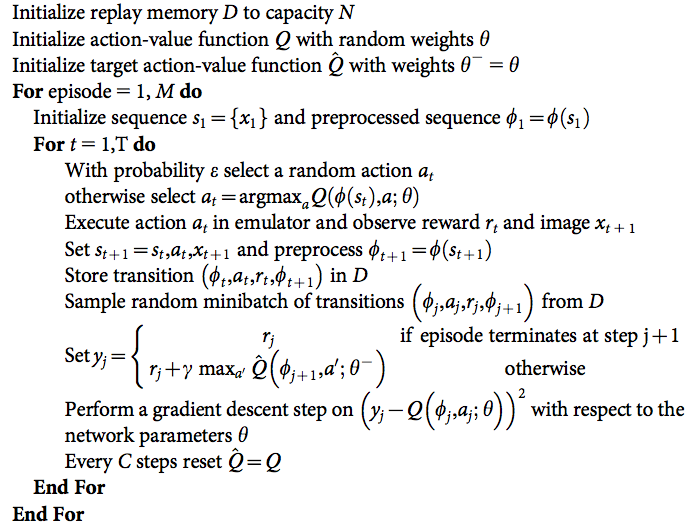There are many extensions of DQN to improve the original design, such as DQN with dueling architecture (Wang et al. 2016) which estimates state-value function V(s) and advantage function A(s, a) with shared network parameters.

## Combining TD and MC Learning#

In the previous section on value estimation in TD learning, we only trace one step further down the action chain when calculating the TD target. One can easily extend it to take multiple steps to estimate the return.

Let’s label the estimated return following n steps as $G_t^{(n)}, n=1, \dots, \infty$, then:

$n$ $G_t$ Notes
$n=1$ $G_t^{(1)} = R_{t+1} + \gamma V(S_{t+1})$ TD learning
$n=2$ $G_t^{(2)} = R_{t+1} + \gamma R_{t+2} + \gamma^2 V(S_{t+2})$
$n=n$ $G_t^{(n)} = R_{t+1} + \gamma R_{t+2} + \dots + \gamma^{n-1} R_{t+n} + \gamma^n V(S_{t+n})$
$n=\infty$ $G_t^{(\infty)} = R_{t+1} + \gamma R_{t+2} + \dots + \gamma^{T-t-1} R_T + \gamma^{T-t} V(S_T)$ MC estimation

The generalized n-step TD learning still has the same form for updating the value function:

$$V(S_t) \leftarrow V(S_t) + \alpha (G_t^{(n)} - V(S_t))$$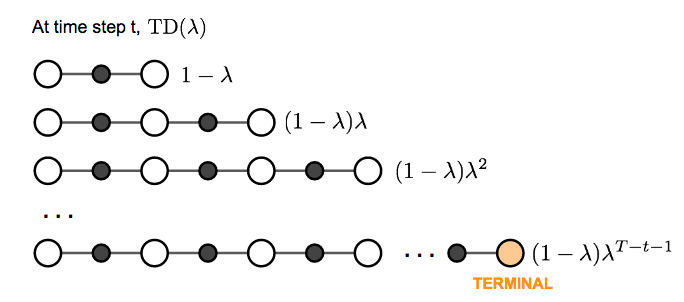We are free to pick any $n$ in TD learning as we like. Now the question becomes what is the best $n$? Which $G_t^{(n)}$ gives us the best return approximation? A common yet smart solution is to apply a weighted sum of all possible n-step TD targets rather than to pick a single best n. The weights decay by a factor λ with n, $\lambda^{n-1}$; the intuition is similar to why we want to discount future rewards when computing the return: the more future we look into the less confident we would be. To make all the weight (n → ∞) sum up to 1, we multiply every weight by (1-λ), because:

\begin{aligned} \text{let } S &= 1 + \lambda + \lambda^2 + \dots \\ S &= 1 + \lambda(1 + \lambda + \lambda^2 + \dots) \\ S &= 1 + \lambda S \\ S &= 1 / (1-\lambda) \end{aligned}

This weighted sum of many n-step returns is called λ-return $G_t^{\lambda} = (1-\lambda) \sum_{n=1}^{\infty} \lambda^{n-1} G_t^{(n)}$. TD learning that adopts λ-return for value updating is labeled as TD(λ). The original version we introduced above is equivalent to TD(0).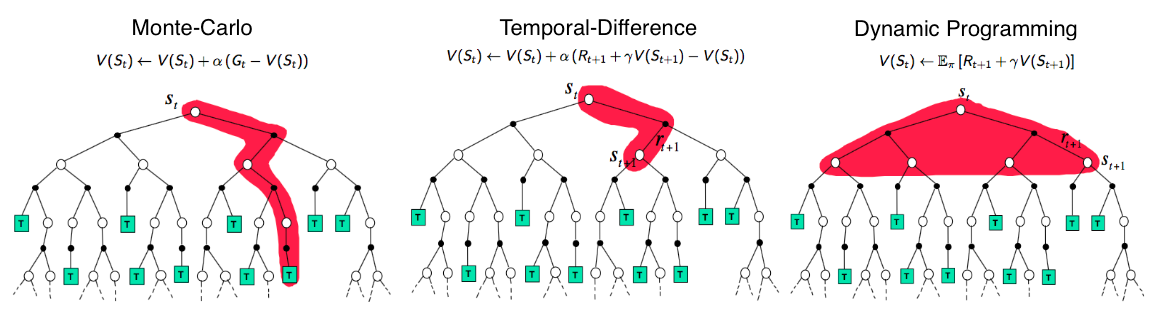All the methods we have introduced above aim to learn the state/action value function and then to select actions accordingly. Policy Gradient methods instead learn the policy directly with a parameterized function respect to $\theta$, $\pi(a \vert s; \theta)$. Let’s define the reward function (opposite of loss function) as the expected return and train the algorithm with the goal to maximize the reward function. My next post described why the policy gradient theorem works (proof) and introduced a number of policy gradient algorithms.

In discrete space:

$$\mathcal{J}(\theta) = V_{\pi_\theta}(S_1) = \mathbb{E}_{\pi_\theta}[V_1]$$

where $S_1$ is the initial starting state.

Or in continuous space:

$$\mathcal{J}(\theta) = \sum_{s \in \mathcal{S}} d_{\pi_\theta}(s) V_{\pi_\theta}(s) = \sum_{s \in \mathcal{S}} \Big( d_{\pi_\theta}(s) \sum_{a \in \mathcal{A}} \pi(a \vert s, \theta) Q_\pi(s, a) \Big)$$

where $d_{\pi_\theta}(s)$ is stationary distribution of Markov chain for $\pi_\theta$. If you are unfamiliar with the definition of a “stationary distribution,” please check this reference.

Using gradient ascent we can find the best θ that produces the highest return. It is natural to expect policy-based methods are more useful in continuous space, because there is an infinite number of actions and/or states to estimate the values for in continuous space and hence value-based approaches are computationally much more expensive.

Computing the gradient numerically can be done by perturbing θ by a small amount ε in the k-th dimension. It works even when $J(\theta)$ is not differentiable (nice!), but unsurprisingly very slow.

$$\frac{\partial \mathcal{J}(\theta)}{\partial \theta_k} \approx \frac{\mathcal{J}(\theta + \epsilon u_k) - \mathcal{J}(\theta)}{\epsilon}$$

Or analytically,

$$\mathcal{J}(\theta) = \mathbb{E}_{\pi_\theta} [r] = \sum_{s \in \mathcal{S}} d_{\pi_\theta}(s) \sum_{a \in \mathcal{A}} \pi(a \vert s; \theta) R(s, a)$$

Actually we have nice theoretical support for (replacing $d(.)$ with $d_\pi(.)$):

$$\mathcal{J}(\theta) = \sum_{s \in \mathcal{S}} d_{\pi_\theta}(s) \sum_{a \in \mathcal{A}} \pi(a \vert s; \theta) Q_\pi(s, a) \propto \sum_{s \in \mathcal{S}} d(s) \sum_{a \in \mathcal{A}} \pi(a \vert s; \theta) Q_\pi(s, a)$$

Check Sec 13.1 in Sutton & Barto (2017) for why this is the case.

Then,

\begin{aligned} \mathcal{J}(\theta) &= \sum_{s \in \mathcal{S}} d(s) \sum_{a \in \mathcal{A}} \pi(a \vert s; \theta) Q_\pi(s, a) \\ \nabla \mathcal{J}(\theta) &= \sum_{s \in \mathcal{S}} d(s) \sum_{a \in \mathcal{A}} \nabla \pi(a \vert s; \theta) Q_\pi(s, a) \\ &= \sum_{s \in \mathcal{S}} d(s) \sum_{a \in \mathcal{A}} \pi(a \vert s; \theta) \frac{\nabla \pi(a \vert s; \theta)}{\pi(a \vert s; \theta)} Q_\pi(s, a) \\ & = \sum_{s \in \mathcal{S}} d(s) \sum_{a \in \mathcal{A}} \pi(a \vert s; \theta) \nabla \ln \pi(a \vert s; \theta) Q_\pi(s, a) \\ & = \mathbb{E}_{\pi_\theta} [\nabla \ln \pi(a \vert s; \theta) Q_\pi(s, a)] \end{aligned}

This result is named “Policy Gradient Theorem” which lays the theoretical foundation for various policy gradient algorithms:

$$\nabla \mathcal{J}(\theta) = \mathbb{E}_{\pi_\theta} [\nabla \ln \pi(a \vert s, \theta) Q_\pi(s, a)]$$

### REINFORCE#

REINFORCE, also known as Monte-Carlo policy gradient, relies on $Q_\pi(s, a)$, an estimated return by MC methods using episode samples, to update the policy parameter $\theta$.

A commonly used variation of REINFORCE is to subtract a baseline value from the return $G_t$ to reduce the variance of gradient estimation while keeping the bias unchanged. For example, a common baseline is state-value, and if applied, we would use $A(s, a) = Q(s, a) - V(s)$ in the gradient ascent update.

1. Initialize θ at random
2. Generate one episode $S_1, A_1, R_2, S_2, A_2, \dots, S_T$
3. For t=1, 2, … , T:
1. Estimate the the return G_t since the time step t.
2. $\theta \leftarrow \theta + \alpha \gamma^t G_t \nabla \ln \pi(A_t \vert S_t, \theta)$.

### Actor-Critic#

If the value function is learned in addition to the policy, we would get Actor-Critic algorithm.

• Critic: updates value function parameters w and depending on the algorithm it could be action-value $Q(a \vert s; w)$ or state-value $V(s; w)$.
• Actor: updates policy parameters θ, in the direction suggested by the critic, $\pi(a \vert s; \theta)$.

Let’s see how it works in an action-value actor-critic algorithm.

1. Initialize s, θ, w at random; sample $a \sim \pi(a \vert s; \theta)$.
2. For t = 1… T:
1. Sample reward $r_t \sim R(s, a)$ and next state $s' \sim P(s' \vert s, a)$.
2. Then sample the next action $a' \sim \pi(s', a'; \theta)$.
3. Update policy parameters: $\theta \leftarrow \theta + \alpha_\theta Q(s, a; w) \nabla_\theta \ln \pi(a \vert s; \theta)$.
4. Compute the correction for action-value at time t:
$G_{t:t+1} = r_t + \gamma Q(s', a'; w) - Q(s, a; w)$
and use it to update value function parameters:
$w \leftarrow w + \alpha_w G_{t:t+1} \nabla_w Q(s, a; w)$.
5. Update $a \leftarrow a'$ and $s \leftarrow s'$.

$\alpha_\theta$ and $\alpha_w$ are two learning rates for policy and value function parameter updates, respectively.

### A3C#

Asynchronous Advantage Actor-Critic (Mnih et al., 2016), short for A3C, is a classic policy gradient method with the special focus on parallel training.

In A3C, the critics learn the state-value function, $V(s; w)$, while multiple actors are trained in parallel and get synced with global parameters from time to time. Hence, A3C is good for parallel training by default, i.e. on one machine with multi-core CPU.

The loss function for state-value is to minimize the mean squared error, $\mathcal{J}_v (w) = (G_t - V(s; w))^2$ and we use gradient descent to find the optimal w. This state-value function is used as the baseline in the policy gradient update.

Here is the algorithm outline:

1. We have global parameters, θ and w; similar thread-specific parameters, θ' and w'.
2. Initialize the time step t = 1
3. While T <= T_MAX:
1. Reset gradient: dθ = 0 and dw = 0.
2. Synchronize thread-specific parameters with global ones: θ' = θ and w' = w.
3. $t_\text{start}$ = t and get $s_t$.
4. While ($s_t \neq \text{TERMINAL}$) and ($t - t_\text{start} <= t_\text{max}$):
1. Pick the action $a_t \sim \pi(a_t \vert s_t; \theta')$ and receive a new reward $r_t$ and a new state $s_{t+1}$.
2. Update t = t + 1 and T = T + 1.
5. Initialize the variable that holds the return estimation $$R = \begin{cases} 0 & \text{if } s_t \text{ is TERMINAL} \ V(s_t; w') & \text{otherwise} \end{cases}$$.
6. For $i = t-1, \dots, t_\text{start}$:
1. $R \leftarrow r_i + \gamma R$; here R is a MC measure of $G_i$.
2. Accumulate gradients w.r.t. θ': $d\theta \leftarrow d\theta + \nabla_{\theta'} \log \pi(a_i \vert s_i; \theta')(R - V(s_i; w'))$;
Accumulate gradients w.r.t. w': $dw \leftarrow dw + \nabla_{w'} (R - V(s_i; w'))^2$.
7. Update synchronously θ using dθ, and w using dw.

A3C enables the parallelism in multiple agent training. The gradient accumulation step (6.2) can be considered as a reformation of minibatch-based stochastic gradient update: the values of w or θ get corrected by a little bit in the direction of each training thread independently.

## Evolution Strategies#

Evolution Strategies (ES) is a type of model-agnostic optimization approach. It learns the optimal solution by imitating Darwin’s theory of the evolution of species by natural selection. Two prerequisites for applying ES: (1) our solutions can freely interact with the environment and see whether they can solve the problem; (2) we are able to compute a fitness score of how good each solution is. We don’t have to know the environment configuration to solve the problem.

Say, we start with a population of random solutions. All of them are capable of interacting with the environment and only candidates with high fitness scores can survive (only the fittest can survive in a competition for limited resources). A new generation is then created by recombining the settings (gene mutation) of high-fitness survivors. This process is repeated until the new solutions are good enough.

Very different from the popular MDP-based approaches as what we have introduced above, ES aims to learn the policy parameter $\theta$ without value approximation. Let’s assume the distribution over the parameter $\theta$ is an isotropic multivariate Gaussian with mean $\mu$ and fixed covariance $\sigma^2I$. The gradient of $F(\theta)$ is calculated:

\begin{aligned} & \nabla_\theta \mathbb{E}_{\theta \sim N(\mu, \sigma^2)} F(\theta) \\ =& \nabla_\theta \int_\theta F(\theta) \Pr(\theta) && \text{Pr(.) is the Gaussian density function.} \\ =& \int_\theta F(\theta) \Pr(\theta) \frac{\nabla_\theta \Pr(\theta)}{\Pr(\theta)} \\ =& \int_\theta F(\theta) \Pr(\theta) \nabla_\theta \log \Pr(\theta) \\ =& \mathbb{E}_{\theta \sim N(\mu, \sigma^2)} [F(\theta) \nabla_\theta \log \Pr(\theta)] && \text{Similar to how we do policy gradient update.} \\ =& \mathbb{E}_{\theta \sim N(\mu, \sigma^2)} \Big[ F(\theta) \nabla_\theta \log \Big( \frac{1}{\sqrt{2\pi\sigma^2}} e^{-\frac{(\theta - \mu)^2}{2 \sigma^2 }} \Big) \Big] \\ =& \mathbb{E}_{\theta \sim N(\mu, \sigma^2)} \Big[ F(\theta) \nabla_\theta \Big( -\log \sqrt{2\pi\sigma^2} - \frac{(\theta - \mu)^2}{2 \sigma^2} \Big) \Big] \\ =& \mathbb{E}_{\theta \sim N(\mu, \sigma^2)} \Big[ F(\theta) \frac{\theta - \mu}{\sigma^2} \Big] \end{aligned}

We can rewrite this formula in terms of a “mean” parameter $\theta$ (different from the $\theta$ above; this $\theta$ is the base gene for further mutation), $\epsilon \sim N(0, I)$ and therefore $\theta + \epsilon \sigma \sim N(\theta, \sigma^2)$. $\epsilon$ controls how much Gaussian noises should be added to create mutation:

$$\nabla_\theta \mathbb{E}_{\epsilon \sim N(0, I)} F(\theta + \sigma \epsilon) = \frac{1}{\sigma} \mathbb{E}_{\epsilon \sim N(0, I)} [F(\theta + \sigma \epsilon) \epsilon]$$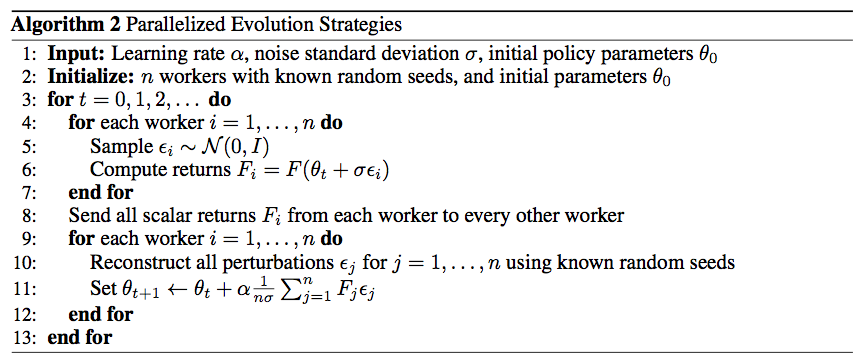ES, as a black-box optimization algorithm, is another approach to RL problems (In my original writing, I used the phrase “a nice alternative”; Seita pointed me to this discussion and thus I updated my wording.). It has a couple of good characteristics (Salimans et al., 2017) keeping it fast and easy to train:

• ES does not need value function approximation;
• ES does not perform gradient back-propagation;
• ES is invariant to delayed or long-term rewards;
• ES is highly parallelizable with very little data communication.

# Known Problems#

## Exploration-Exploitation Dilemma#

The problem of exploration vs exploitation dilemma has been discussed in my previous post. When the RL problem faces an unknown environment, this issue is especially a key to finding a good solution: without enough exploration, we cannot learn the environment well enough; without enough exploitation, we cannot complete our reward optimization task.

Different RL algorithms balance between exploration and exploitation in different ways. In MC methods, Q-learning or many on-policy algorithms, the exploration is commonly implemented by ε-greedy; In ES, the exploration is captured by the policy parameter perturbation. Please keep this into consideration when develop a new RL algorithm.

We do seek the efficiency and flexibility of TD methods that involve bootstrapping. However, when off-policy, nonlinear function approximation, and bootstrapping are combined in one RL algorithm, the training could be unstable and hard to converge. This issue is known as the deadly triad (Sutton & Barto, 2017). Many architectures using deep learning models were proposed to resolve the problem, including DQN to stabilize the training with experience replay and occasionally frozen target network.

# Case Study: AlphaGo Zero#

The game of Go has been an extremely hard problem in the field of Artificial Intelligence for decades until recent years. AlphaGo and AlphaGo Zero are two programs developed by a team at DeepMind. Both involve deep Convolutional Neural Networks (CNN) and Monte Carlo Tree Search (MCTS) and both have been approved to achieve the level of professional human Go players. Different from AlphaGo that relied on supervised learning from expert human moves, AlphaGo Zero used only reinforcement learning and self-play without human knowledge beyond the basic rules.With all the knowledge of RL above, let’s take a look at how AlphaGo Zero works. The main component is a deep CNN over the game board configuration (precisely, a ResNet with batch normalization and ReLU). This network outputs two values:

$$(p, v) = f_\theta(s)$$
• $s$: the game board configuration, 19 x 19 x 17 stacked feature planes; 17 features for each position, 8 past configurations (including current) for the current player + 8 past configurations for the opponent + 1 feature indicating the color (1=black, 0=white). We need to code the color specifically because the network is playing with itself and the colors of current player and opponents are switching between steps.
• $p$: the probability of selecting a move over 19^2 + 1 candidates (19^2 positions on the board, in addition to passing).
• $v$: the winning probability given the current setting.

During self-play, MCTS further improves the action probability distribution $\pi \sim p(.)$ and then the action $a_t$ is sampled from this improved policy. The reward $z_t$ is a binary value indicating whether the current player eventually wins the game. Each move generates an episode tuple $(s_t, \pi_t, z_t)$ and it is saved into the replay memory. The details on MCTS are skipped for the sake of space in this post; please read the original paper if you are interested.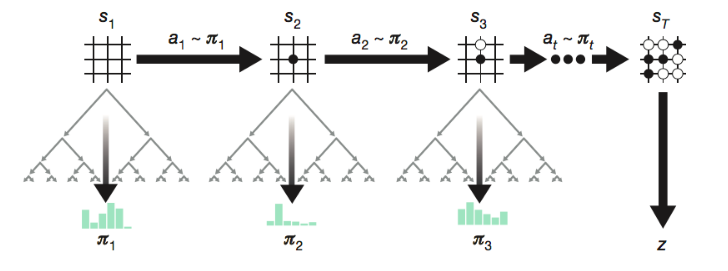The network is trained with the samples in the replay memory to minimize the loss:

$$\mathcal{L} = (z - v)^2 - \pi^\top \log p + c \| \theta \|^2$$

where $c$ is a hyperparameter controlling the intensity of L2 penalty to avoid overfitting.

AlphaGo Zero simplified AlphaGo by removing supervised learning and merging separated policy and value networks into one. It turns out that AlphaGo Zero achieved largely improved performance with a much shorter training time! I strongly recommend reading these two papers side by side and compare the difference, super fun.

I know this is a long read, but hopefully worth it. If you notice mistakes and errors in this post, don’t hesitate to contact me at [lilian dot wengweng at gmail dot com]. See you in the next post! :)

Cited as:

@article{weng2018bandit,
title   = "A (Long) Peek into Reinforcement Learning",
author  = "Weng, Lilian",
journal = "lilianweng.github.io",
year    = "2018",
url     = "https://lilianweng.github.io/posts/2018-02-19-rl-overview/"
}


 Yuxi Li. Deep reinforcement learning: An overview. arXiv preprint arXiv:1701.07274. 2017.

 Richard S. Sutton and Andrew G. Barto. Reinforcement Learning: An Introduction; 2nd Edition. 2017.

 Volodymyr Mnih, et al. Asynchronous methods for deep reinforcement learning. ICML. 2016.

 Tim Salimans, et al. Evolution strategies as a scalable alternative to reinforcement learning. arXiv preprint arXiv:1703.03864 (2017).

 David Silver, et al. Mastering the game of go without human knowledge. Nature 550.7676 (2017): 354.

 David Silver, et al. Mastering the game of Go with deep neural networks and tree search. Nature 529.7587 (2016): 484-489.

 Volodymyr Mnih, et al. Human-level control through deep reinforcement learning. Nature 518.7540 (2015): 529.

 Ziyu Wang, et al. Dueling network architectures for deep reinforcement learning. ICML. 2016.

 Reinforcement Learning lectures by David Silver on YouTube.

 Frank Sehnke, et al. Parameter-exploring policy gradients. Neural Networks 23.4 (2010): 551-559.

 Csaba Szepesvári. Algorithms for reinforcement learning. 1st Edition. Synthesis lectures on artificial intelligence and machine learning 4.1 (2010): 1-103.

If you notice mistakes and errors in this post, please don’t hesitate to contact me at [lilian dot wengweng at gmail dot com] and I would be super happy to correct them right away!